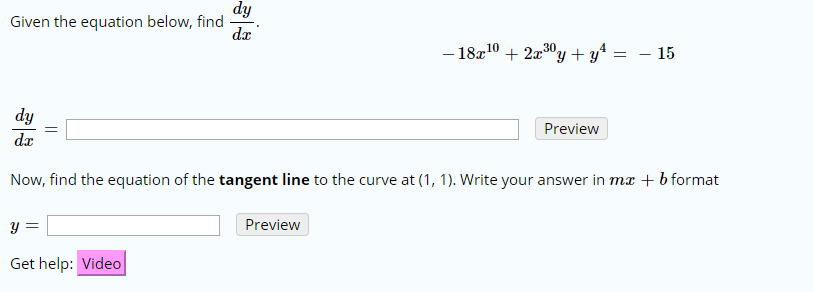# dy Given the equation below, find da -18102x30y + y* 4 - 15 dy Preview da Now, find the equation of the tangent line to the curve at (1, 1). Write your answer in mx b format Preview Get help: Video

Questionhelp_outlineImage Transcriptionclosedy Given the equation below, find da -18102x30y + y* 4 - 15 dy Preview da Now, find the equation of the tangent line to the curve at (1, 1). Write your answer in mx b format Preview Get help: Video fullscreen